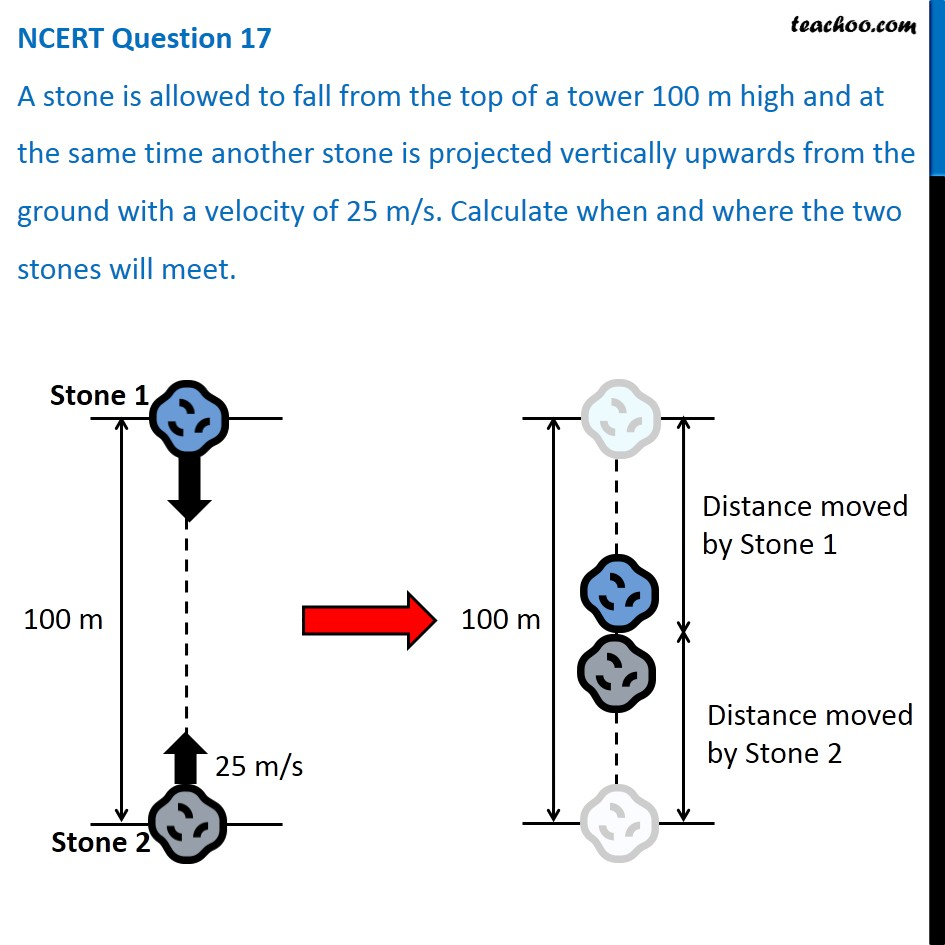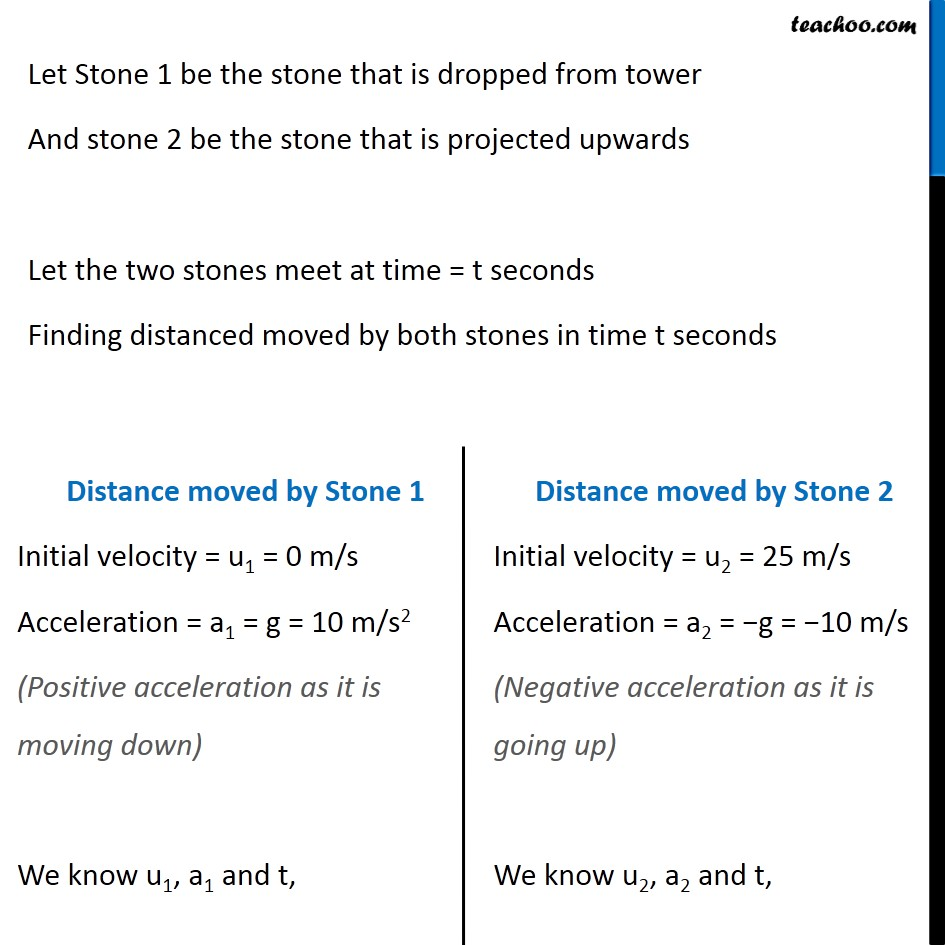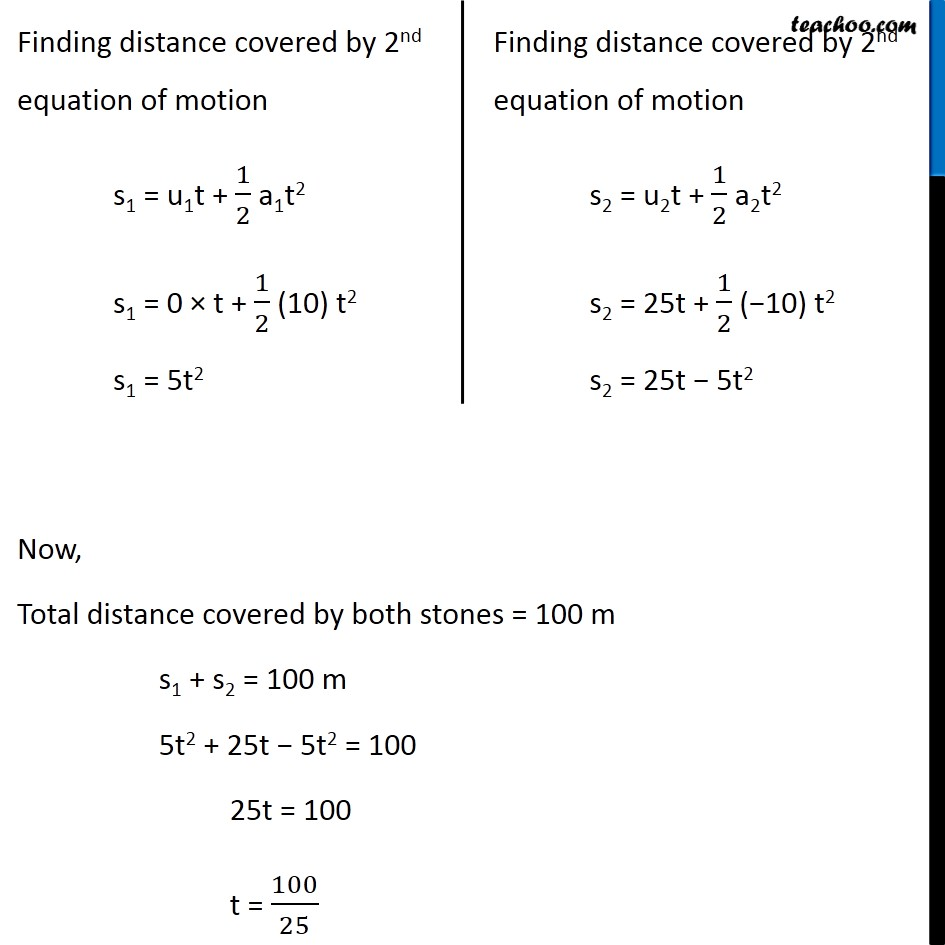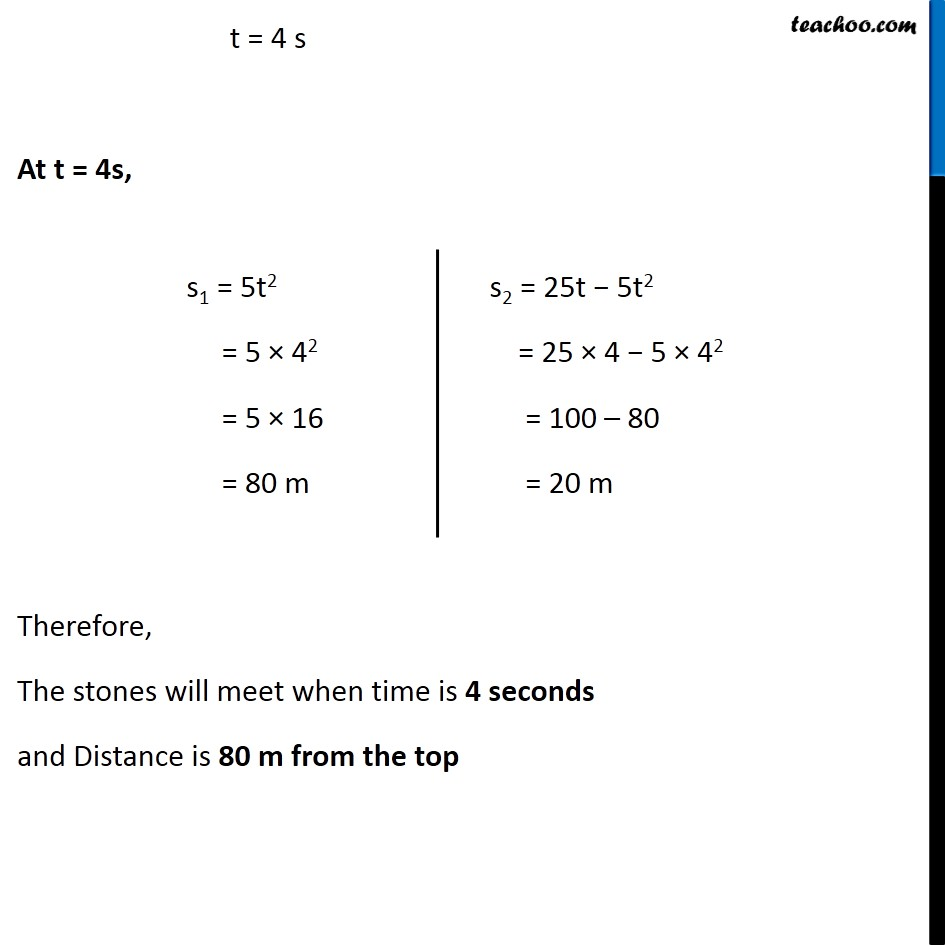NCERT Questions

Class 9
Chapter 10 Class 9 - GravitationGet live Maths 1-on-1 Classs - Class 6 to 12

### Transcript

NCERT Question 17 A stone is allowed to fall from the top of a tower 100 m high and at the same time another stone is projected vertically upwards from the ground with a velocity of 25 m/s. Calculate when and where the two stones will meet. Distance moved by Stone 1 Distance moved by Stone 2 Let Stone 1 be the stone that is dropped from tower And stone 2 be the stone that is projected upwards Let the two stones meet at time = t seconds Finding distanced moved by both stones in time t seconds Distance moved by Stone 1 Initial velocity = u1 = 0 m/s Acceleration = a1 = g = 10 m/s2 (Positive acceleration as it is moving down) We know u1, a1 and t, Distance moved by Stone 2 Initial velocity = u2 = 25 m/s Acceleration = a2 = −g = −10 m/s (Negative acceleration as it is going up) We know u2, a2 and t, Finding distance covered by 2nd equation of motion s1 = u1t + 1/2 a1t2 s1 = 0 × t + 1/2 (10) t2 s1 = 5t2 Finding distance covered by 2nd equation of motion s2 = u2t + 1/2 a2t2 s2 = 25t + 1/2 (−10) t2 s2 = 25t − 5t2 Now, Total distance covered by both stones = 100 m s1 + s2 = 100 m 5t2 + 25t − 5t2 = 100 25t = 100 t = 100/25 t = 4 s At t = 4s, s1 = 5t2 = 5 × 42 = 5 × 16 = 80 m s2 = 25t − 5t2 = 25 × 4 − 5 × 42 = 100 – 80 = 20 m Therefore, The stones will meet when time is 4 seconds and Distance is 80 m from the top